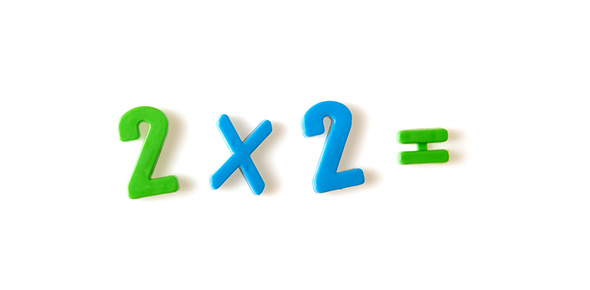# Math Multiplication (1-digit Number)

10 Questions | Total Attempts: 47SettingsCheck out this math multiplication practice for your kids!

• 1.
9 x 5 =
• A.

55

• B.

45

• C.

46

• D.

37

• 2.
8 x 9 =
• A.

62

• B.

53

• C.

71

• D.

72

• 3.
9 x 5 =
• A.

44

• B.

55

• C.

45

• D.

36

• 4.
7 x 1 =
• A.

7

• B.

9

• C.

5

• D.

10

• 5.
5 x 1 =
• A.

6

• B.

8

• C.

5

• D.

13

• 6.
3 x 2 =
• A.

5

• B.

8

• C.

7

• D.

6

• 7.
4 x 3 =
• A.

11

• B.

12

• C.

15

• D.

21

• 8.
7 x 5 =
• A.

34

• B.

25

• C.

16

• D.

35

• 9.
9 x 6 =
• A.

28

• B.

53

• C.

54

• D.

60

• 10.
8 x 4 =
• A.

32

• B.

31

• C.

45

• D.

53

Related Topics